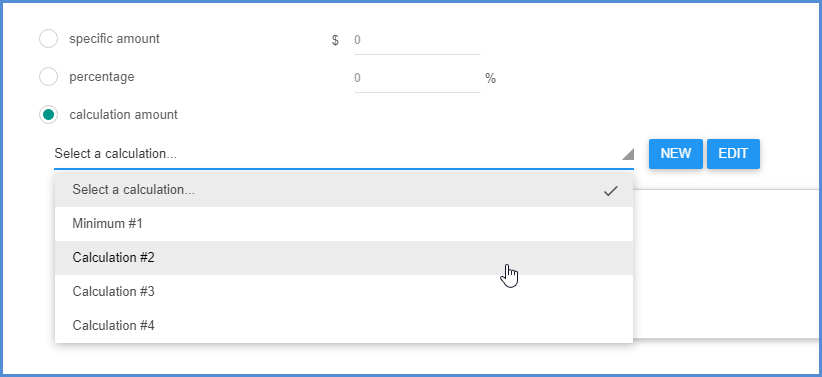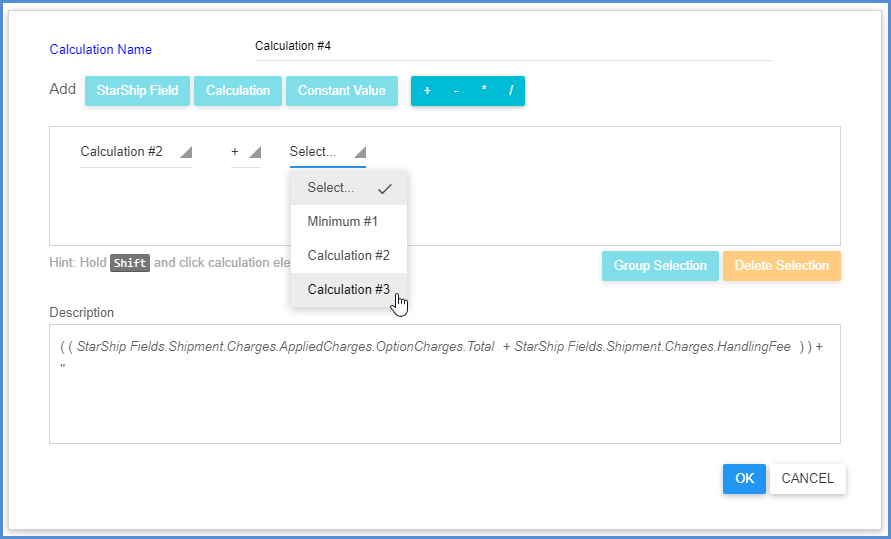1. Home
2. Shipping
3. Freight Rules
4. Freight Rules Calculations
1. Home
2. StarShip 101
3. Freight Rules
4. Freight Rules Calculations

# Freight Rules Calculations

StarShip gives you the option to create calculations when adding freight rules so that you can set the charges to the result of a calculation. You can define a calculation once and save it so that you can reuse the same calculation and select it for a different freight rule. Saved calculations are available in a drop-down list.Calculations can be created for the Min/Max, Increase, Decrease, and Calculation types of freight rules.

### New Calculation

1. From within the freight rules wizard, choose the calculation option and click New.
2. Type in a Calculation Name or accept the default name.
3. Next, create the calculation. You can add a StarShip field, Calculation (previously created) or Constant Value.
4. Continue to build the calculation by adding an operator (multiply, divide, add, or subtract the values) and a second StarShip field, Calculation or Constant Value. As you add elements, the expanded calculation displays in the bottom pane.### Grouping Elements

To group calculation elements, press the Shift key and click on the field values that you want to contain in one expression. Then, click the Group Selection button.Groups are denoted by parentheses.### Complex Calculations

You can use an existing calculation within another one to create more complex calculations. To select existing calculations, click the Calculation button and choose from the drop-down list.The complete complex calculation displays in the Description field.